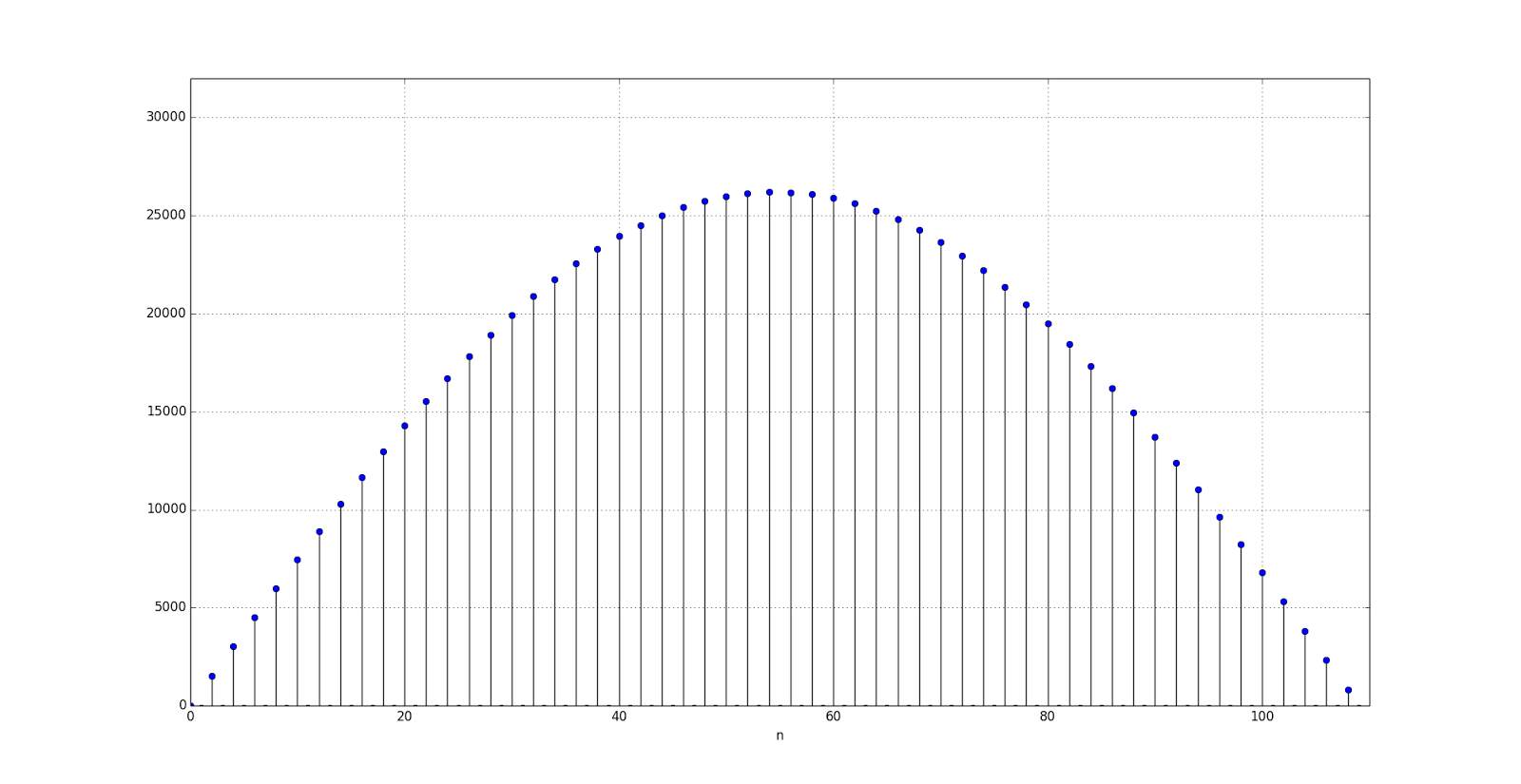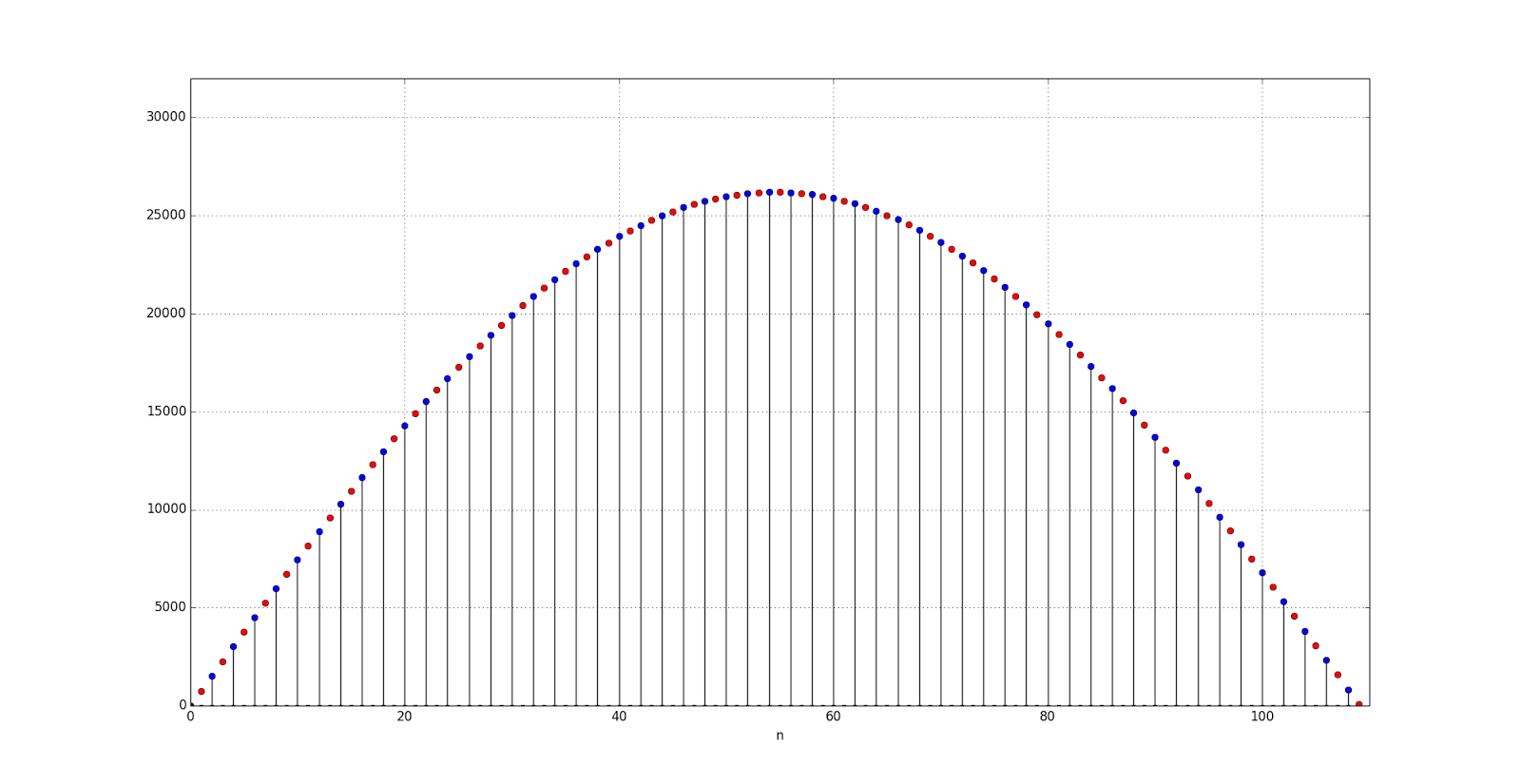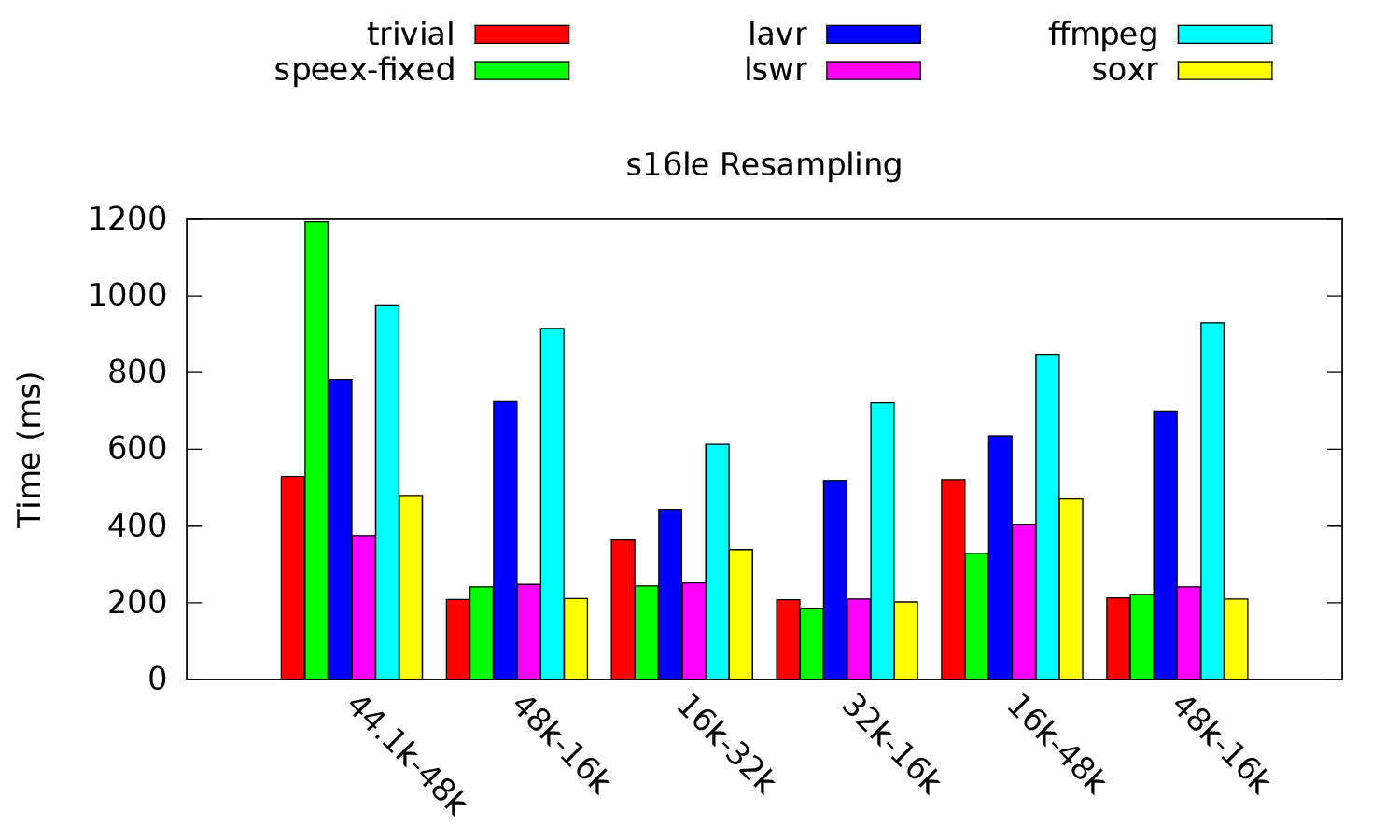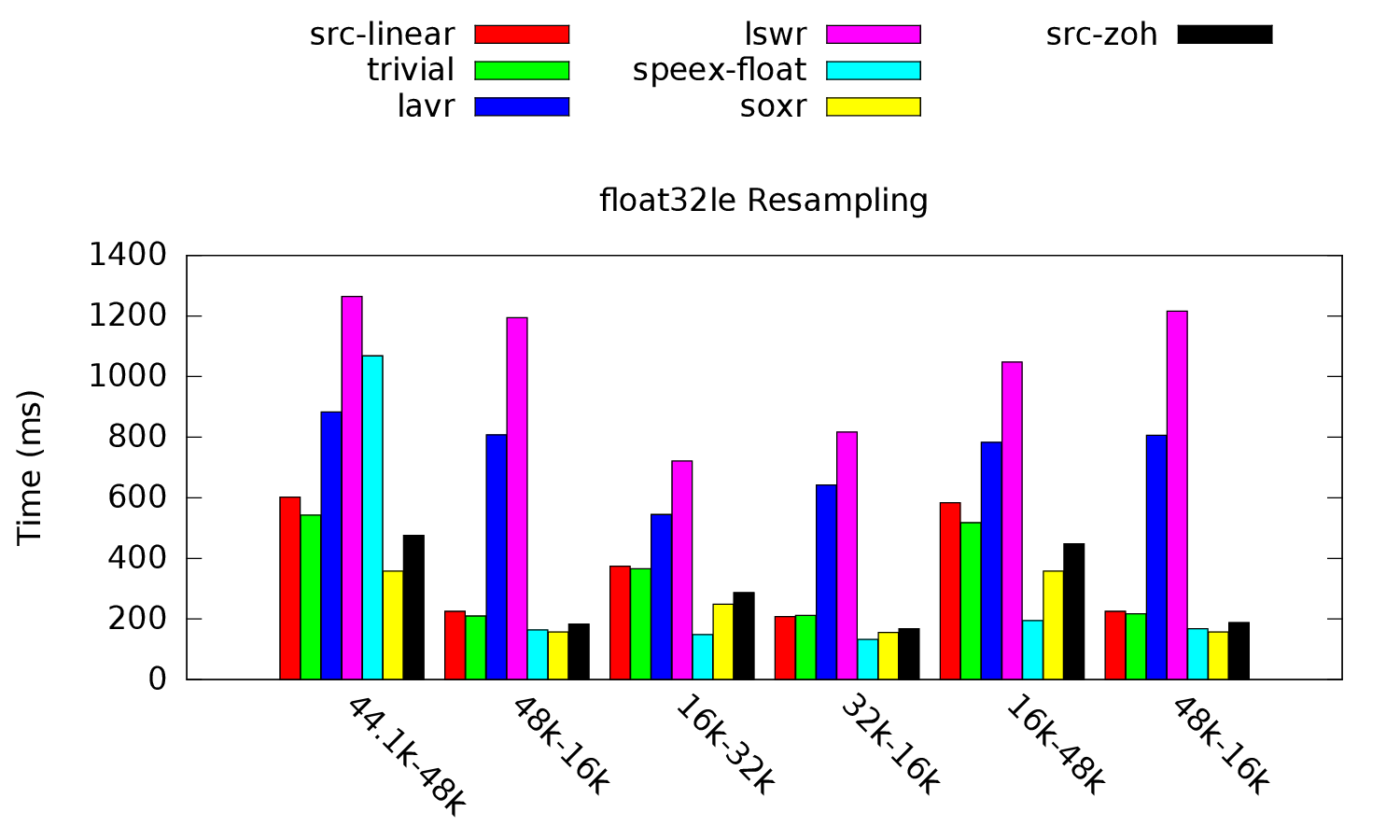## Monday, August 12, 2013

### Vol 2: Resampling methods

Hi.
Time for an update. This time I will talk a little bit about the different resampling methods and resampling in general. So lets start with a quick introduction to resampling.

Below is a figure of a discrete time representation of a 440Hz sine wave. The sine wave is sampled at a rate (or sampling frequency) of 48kHz. This means that roughly every 0.02ms a sample of our sine wave is taken so for one second of sound 48000 samples are needed.440Hz sine wave sampled at 48kHz

Now if the clock in our sound card supports this frequency, we can just feed it our sine wave and it will play it just fine. If this is not the case, we would get pitch shifted output just like when the playback speed on an old tape recorder or turntable is increased or decreased.

Conceptually, we could reconstruct our analog signal and sample it at our new sample rate to obtain our desired discrete time representation. But this is not a really practical solution and we use pure mathematical solutions instead. One of them is linear interpolation. Shown below is our original sine wave resampled via linear interpolation to 96kHz which means that we now have twice as many samples than in our original sampled sine wave.440Hz sine wave resampled to 96kHz
There are many different resampling methods and implementations. PulseAudio already supports quite a few. I added support for some more and tested their performance.

Here are the newly added methods:
• libswresample (lswr below)
• libavresample (lavr below)
• sox resampler (soxr below)
And here are the test results:Performance using signed 16-bit integers as the sample format

These results should be taken with a grain of salt because the different resampling methods do not carry the same quality. The most interesting resampler here seems to be soxr using cubic interpolation.

Below is the same test but this time using floating point numbers as the sample format:Performance using floating point numbers as the sample format

Again soxr here seems to be the most promising.

Which of these new resampling methods will find their way into the master tree of PulseAudio remains to be seen.

This ended up somewhat longer than anticipated, but I hope it was interesting.